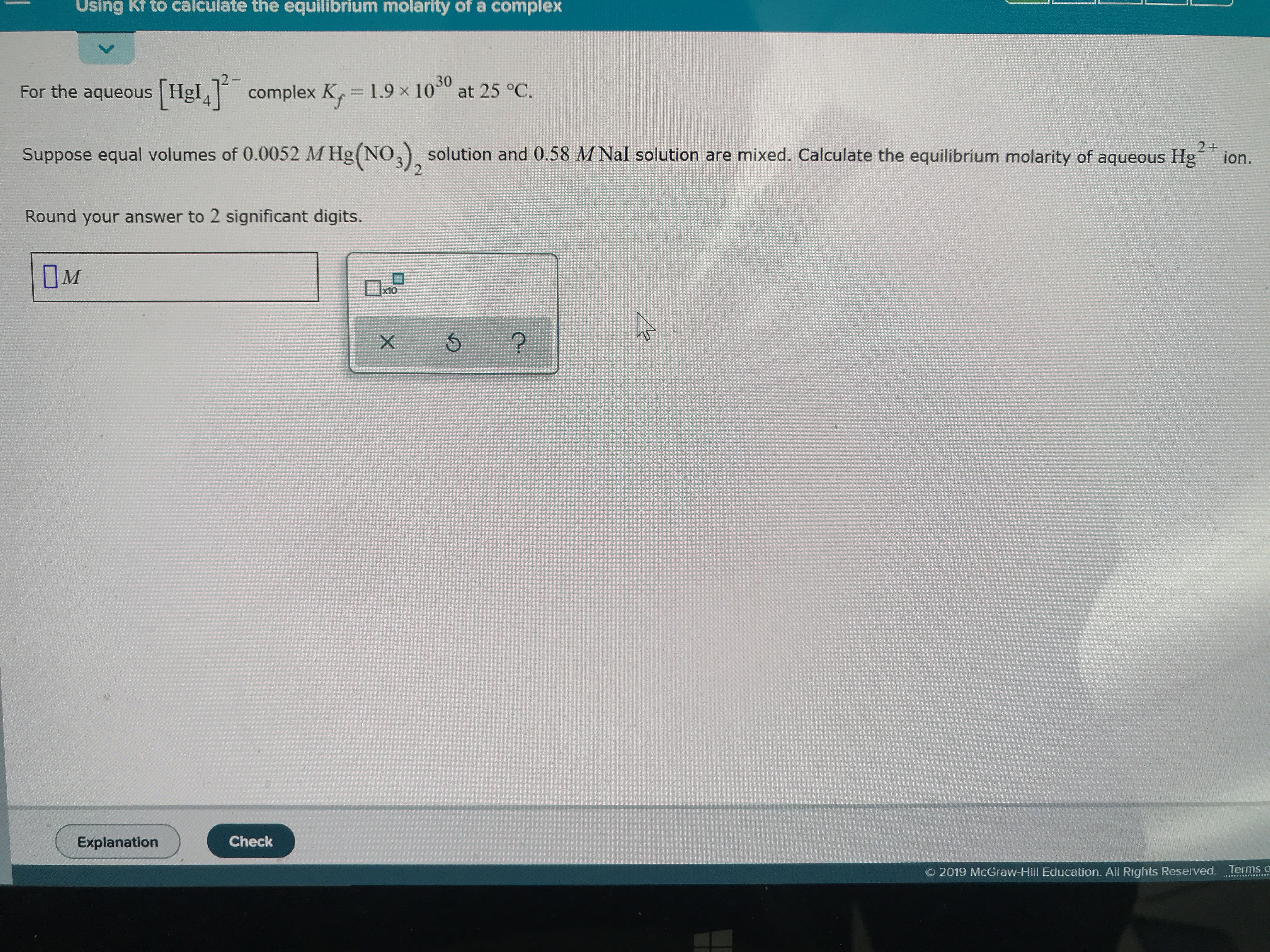# For the aqueous [HgI4]2- complex Kf=1.9x10^30 at 25C. Suppose equal volumes of 0.0052 M Hg(NO3)2 solution and 0.58 M NaI solution are mixed. Calculate the equilibrium molarity of aqueous Hg2+. Round to two significant digits